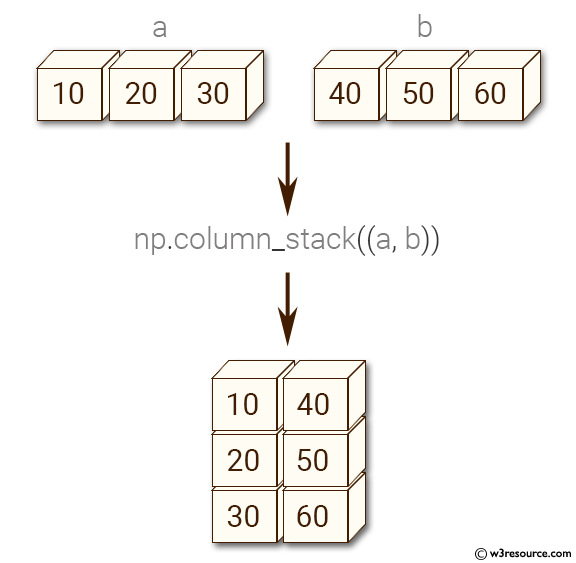﻿ NumPy: Convert 1-D arrays as columns into a 2-D array - w3resource# NumPy: Convert 1-D arrays as columns into a 2-D array

## NumPy: Array Object Exercise-59 with Solution

Write a NumPy program to convert 1-D arrays as columns into a 2-D array.
Sample array: (10,20,30), (40,50,60)

Pictorial Presentation:Sample Solution:-

Python Code:

``````import numpy as np
a = np.array((10,20,30))
b = np.array((40,50,60))
c = np.column_stack((a, b))
print(c)
```
```

Sample Output:

```[[10 40]
[20 50]
[30 60]]
```

Python Code Editor:

Have another way to solve this solution? Contribute your code (and comments) through Disqus.

What is the difficulty level of this exercise?

Test your Python skills with w3resource's quiz

﻿

## Python: Tips of the Day

Set comprehension:

```>>> m = {x ** 2 for x in range(5)}
>>> m
{0, 1, 4, 9, 16}
```